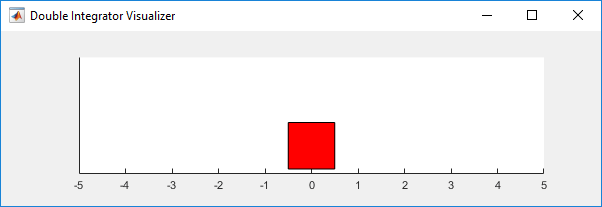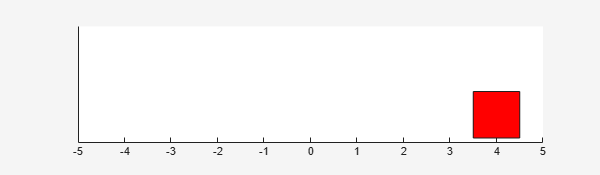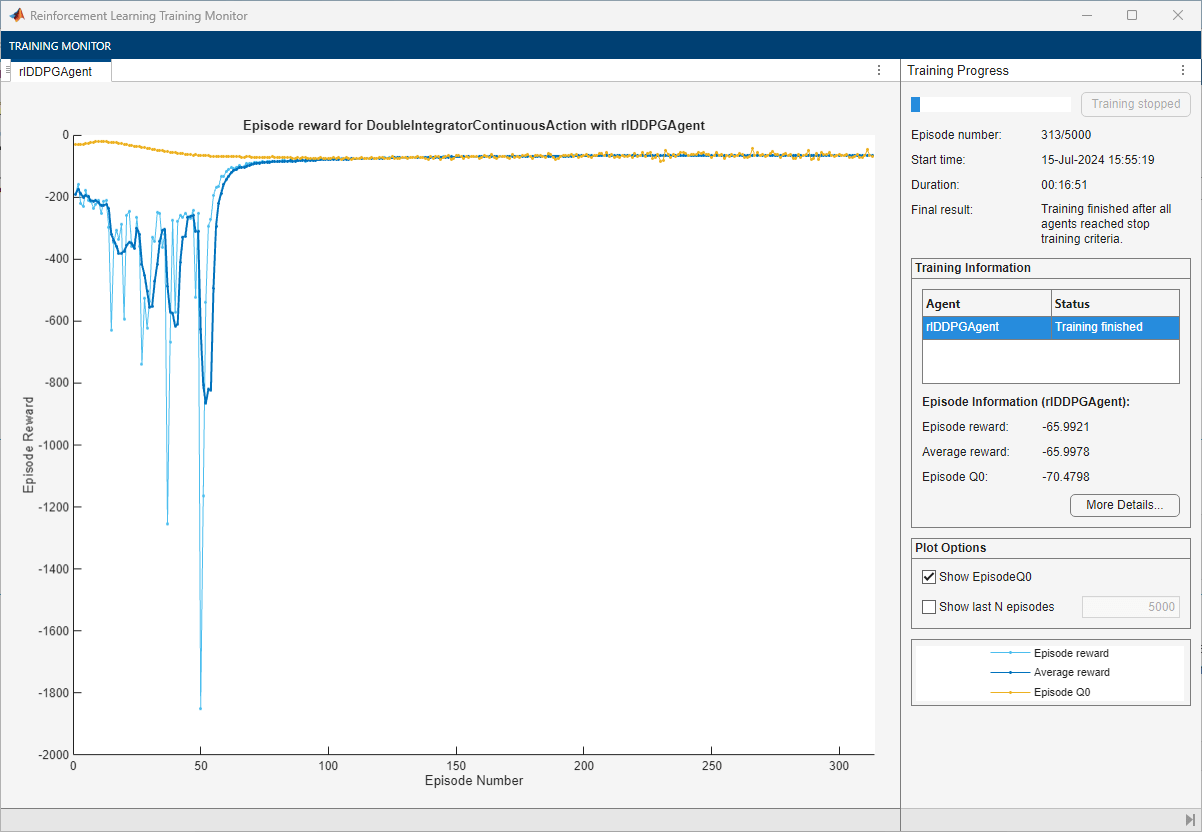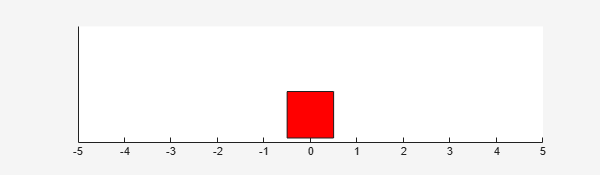# Train DDPG Agent to Control Double Integrator System

This example shows how to train a deep deterministic policy gradient (DDPG) agent to control a second-order linear dynamic system modeled in MATLAB®. The example also compares the DDPG agent to an LQR controller.

For more information on DDPG agents, see Deep Deterministic Policy Gradient (DDPG) Agents. For an example showing how to train a DDPG agent in Simulink®, see Train DDPG Agent to Swing Up and Balance Pendulum.

### Double Integrator MATLAB Environment

The reinforcement learning environment for this example is a second-order double-integrator system with a gain. The training goal is to control the position of a mass in the second-order system by applying a force input.For this environment:

• The mass starts at an initial position between –4 and 4 units.

• The observations from the environment are the position and velocity of the mass.

• The episode terminates if the mass moves more than 5 m from the original position or if $|\mathit{x}|<0.01$.

• The reward ${\mathit{r}}_{\mathit{t}}$, provided at every time step, is a discretization of $\mathit{r}\left(\mathit{t}\right)$:

• $\mathit{r}\left(\mathit{t}\right)=-\left({\mathit{x}\left(\mathit{t}\right)}^{\prime }\text{\hspace{0.17em}}\mathit{Q}\text{\hspace{0.17em}}\mathit{x}\left(\mathit{t}\right)+{\mathit{u}\left(\mathit{t}\right)}^{\prime }\text{\hspace{0.17em}}\mathit{R}\text{\hspace{0.17em}}\mathit{u}\left(\mathit{t}\right)\right)$

Here:

• $\mathit{x}$ is the state vector of the mass.

• $\mathit{u}$ is the force applied to the mass.

• $\mathit{Q}$ is the matrix of weights on the control performance; $\mathit{Q}=\left[10\text{\hspace{0.17em}}0;0\text{\hspace{0.17em}}1\right]$.

• $\mathit{R}$ is the weight on the control effort; $\mathit{R}=0.01$.

For this example the environment is a linear dynamical system, the environment state is observed directly, and the reward is a quadratic function of the observation and action. Therefore the problem of finding the sequence of actions that minimizes the cumulative long-term reward is a discrete-time linear-quadratic optimal control problem, for which the optimal action is known to be a linear function of the system states. This problem can also be solved using Linear-Quadratic Regulator (LQR) design, and in the last part of the example you can compare the agent to an LQR controller.

### Create Environment Interface

Create a predefined environment interface for the double integrator system.

`env = rlPredefinedEnv("DoubleIntegrator-Continuous")`
```env = DoubleIntegratorContinuousAction with properties: Gain: 1 Ts: 0.1000 MaxDistance: 5 GoalThreshold: 0.0100 Q: [2x2 double] R: 0.0100 MaxForce: Inf State: [2x1 double] ```

The interface has a continuous action space where the agent can apply force values from -`Inf` to `Inf` to the mass. The sample time is stored in `env.Ts`, while the continuous time cost function matrices are stored in `env.Q` and `env.R` respectively.

Obtain the observation and action information from the environment interface.

`obsInfo = getObservationInfo(env)`
```obsInfo = rlNumericSpec with properties: LowerLimit: -Inf UpperLimit: Inf Name: "states" Description: "x, dx" Dimension: [2 1] DataType: "double" ```
`actInfo = getActionInfo(env)`
```actInfo = rlNumericSpec with properties: LowerLimit: -Inf UpperLimit: Inf Name: "force" Description: [0x0 string] Dimension: [1 1] DataType: "double" ```

Reset the environment and get its initial state.

`x0 = reset(env)`
```x0 = 2×1 4 0 ```

Fix the random generator seed for reproducibility.

`rng(0)`

### Create DDPG Agent

A DDPG agent approximates the discounted cumulative long-term reward using a Q-value-function critic. A Q-value function critic must accept an observation and an action as inputs and return a scalar (the estimated discounted cumulative long-term reward) as output. To approximate the Q-value function within the critic, use a neural network. The value function of the optimal policy is known to be quadratic, use a network with a quadratic layer (which outputs a vector of quadratic monomials, as described in `quadraticLayer`) and a fully connected layer (which provides a linear combination of its inputs).

Define each network path as an array of layer objects and get the dimension of the observation and action spaces from the environment specification objects. Assign names to the network input layers, so you can connect them to the output path and later explicitly associate them with the appropriate environment channel. Since there is no need for a bias term, set the bias term to zero (`Bias=0`) and prevent it from changing (`BiasLearnRateFactor=0`).

For more information on creating value function approximators, see Create Policies and Value Functions.

```% Observation and action paths obsPath = featureInputLayer(obsInfo.Dimension(1),Name="obsIn"); actPath = featureInputLayer(actInfo.Dimension(1),Name="actIn"); % Common path commonPath = [ concatenationLayer(1,2,Name="concat") quadraticLayer fullyConnectedLayer(1,Name="value", ... BiasLearnRateFactor=0,Bias=0) ]; % Add layers to layerGraph object criticNet = layerGraph(obsPath); criticNet = addLayers(criticNet,actPath); criticNet = addLayers(criticNet,commonPath); % Connect layers criticNet = connectLayers(criticNet,"obsIn","concat/in1"); criticNet = connectLayers(criticNet,"actIn","concat/in2");```

View the critic network configuration.

```figure plot(criticNet)```Convert to `dlnetwork` and display the number of weights.

```criticNet = dlnetwork(criticNet); summary(criticNet)```
``` Initialized: true Number of learnables: 7 Inputs: 1 'obsIn' 2 features 2 'actIn' 1 features ```

Create the critic approximator object using `criticNet`, the environment observation and action specifications, and the names of the network input layers to be connected with the environment observation and action channels, respectively. For more information, see `rlQValueFunction`.

```critic = rlQValueFunction(criticNet, ... obsInfo,actInfo, ... ObservationInputNames="obsIn",ActionInputNames="actIn");```

Check the critic with a random observation and action input.

`getValue(critic,{rand(obsInfo.Dimension)},{rand(actInfo.Dimension)})`
```ans = single -0.3977 ```

DDPG agents use a parametrized continuous deterministic policy, which is implemented by a continuous deterministic actor. This actor must accept an observation as input and return an action as output. To approximate the policy function within the actor, use a neural network. Since for this example the optimal policy is known to be linear in the state, use a shallow network with a fully connected layer to provide a linear combination of the two network inputs.

Define the network as an array of layer objects, and get the dimension of the observation and action spaces from the environment specification objects. Since there is no need for a bias term, as done for the critic, set the bias term to zero (`Bias=0`) and prevent it from changing (`BiasLearnRateFactor=0`). For more information on actors, see Create Policies and Value Functions.

```actorNet = [ featureInputLayer(obsInfo.Dimension(1)) fullyConnectedLayer(actInfo.Dimension(1), ... BiasLearnRateFactor=0,Bias=0) ];```

Convert to `dlnetwork` and display the number of weights.

```actorNet = dlnetwork(actorNet); summary(actorNet)```
``` Initialized: true Number of learnables: 3 Inputs: 1 'input' 2 features ```

Create the actor using `actorNet` and the observation and action specifications. For more information, see `rlContinuousDeterministicActor`.

`actor = rlContinuousDeterministicActor(actorNet,obsInfo,actInfo);`

Check the actor with a random observation input.

`getAction(actor,{rand(obsInfo.Dimension)})`
```ans = 1x1 cell array {[0.3493]} ```

Create the DDPG agent using the actor and critic. For more information, see `rlDDPGAgent`.

`agent = rlDDPGAgent(actor,critic);`

Specify options for the agent, including training options for the critic, using dot notation. Alternatively, you can use `rlDDPGAgentOptions`, and `rlOptimizerOptions` objects before creating the agent.

```agent.AgentOptions.SampleTime = env.Ts; agent.AgentOptions.ExperienceBufferLength = 1e6; agent.AgentOptions.MiniBatchSize = 32; agent.AgentOptions.NoiseOptions.Variance = 0.3; agent.AgentOptions.NoiseOptions.VarianceDecayRate = 1e-7; agent.AgentOptions.ActorOptimizerOptions.LearnRate = 1e-4; agent.AgentOptions.ActorOptimizerOptions.GradientThreshold = 1; agent.AgentOptions.CriticOptimizerOptions.LearnRate = 5e-3; agent.AgentOptions.CriticOptimizerOptions.GradientThreshold = 1;```

### Initialize Agent Parameters

The policy implemented by the actor is $\mathit{u}={\mathit{K}}_{1}{\mathit{x}}_{1}+{\mathit{K}}_{2}{\mathit{x}}_{2}=\mathit{Kx}$, where the feedback gains ${\mathit{K}}_{1}$ and ${\mathit{K}}_{2}$ are the two weights of the actor network. It can be shown that the closed loop system is stable if these gains are negative, therefore, initializing them to negative values can speed up convergence.

The Q-value function has the following structure:

`$\mathit{Q}\left(\mathit{x},\mathit{u}\right)={\mathit{W}}_{1}{\mathit{x}}_{1}^{2}+{\mathit{W}}_{2}{\text{\hspace{0.17em}}\mathit{x}}_{1}\text{\hspace{0.17em}}{\mathit{x}}_{2}+{\mathit{W}}_{3}\text{\hspace{0.17em}}{\mathit{x}}_{2}^{2}+{\mathit{W}}_{4}\text{\hspace{0.17em}}{\mathit{x}}_{1}\text{\hspace{0.17em}}\mathit{u}+{\mathit{W}}_{5}{\text{\hspace{0.17em}}\mathit{x}}_{2}\text{\hspace{0.17em}}\mathit{u}\text{\hspace{0.17em}}+{\mathit{W}}_{6}\text{\hspace{0.17em}}{\mathit{u}}_{}^{2}$`

Where${\mathit{W}}_{\mathit{i}}$ are the weights of the fully connected layer. Alternatively, in matrix form:

`$\mathit{Q}\left(\mathit{x},\mathit{u}\right)=\left[\begin{array}{ccc}{\mathit{x}}_{1}& {\mathit{x}}_{2}& \mathit{u}\end{array}\right]\text{\hspace{0.17em}}\left[\begin{array}{ccc}{\mathit{W}}_{1}& \frac{{\mathit{W}}_{2}}{2}& \frac{{\mathit{W}}_{4}}{2}\\ \frac{{\mathit{W}}_{2}}{2}& {\mathit{W}}_{3}& \frac{{\mathit{W}}_{5}}{2}\\ \frac{{\mathit{W}}_{4}}{2}& \frac{{\mathit{W}}_{5}}{2}& {\mathit{W}}_{6}\end{array}\right]\text{\hspace{0.17em}}\left[\begin{array}{c}{\mathit{x}}_{1}\\ {\mathit{x}}_{2}\\ \mathit{u}\end{array}\right]=\left[\begin{array}{cc}{\mathit{x}}^{\mathit{T}}& \mathit{u}\end{array}\right]\mathit{W}\left[\begin{array}{c}\mathit{x}\\ \mathit{u}\end{array}\right]$`

For a fixed policy $\mathit{u}=\mathit{Kx}$, the cumulative long-term reward (that is the value of the policy) becomes:

`$\mathit{V}\left(\mathit{x}\right)=\mathit{Q}\left(\mathit{x},\mathit{Kx}\right)=$`

`${\mathit{x}}^{\mathit{T}}\text{\hspace{0.17em}}\left[\begin{array}{cc}\mathit{I}& {\mathit{K}}^{\mathit{T}}\end{array}\right]\mathit{W}\left[\begin{array}{c}\mathit{I}\\ \mathit{K}\end{array}\right]\text{\hspace{0.17em}}\mathit{x}=$`

`${\mathit{x}}^{\mathit{T}}\mathit{Px}$`

Since the rewards are always negative, to properly approximate the cumulative reward both $\mathit{P}$ and $\mathit{W}$ must be negative definite. Therefore, to speed up convergence, initialize the critic network weights${\mathit{W}}_{\mathit{i}}$ so that $\mathit{W}$ is negative definite.

```% Create diagonal matrix with negative eigenvalues W = -single(diag([1 1 1])+0.1)```
```W = 3x3 single matrix -1.1000 -0.1000 -0.1000 -0.1000 -1.1000 -0.1000 -0.1000 -0.1000 -1.1000 ```
```% Extract indexes of upper triangular part of a 3 by 3 matrix idx = triu(true(3))```
```idx = 3x3 logical array 1 1 1 0 1 1 0 0 1 ```
```% Update parameters in the actor and critic par = getLearnableParameters(agent); par.Actor{1} = -single([1 1]); par.Critic{1} = W(idx)'; setLearnableParameters(agent,par);```

Check the agent with a random observation input.

`getAction(agent,{rand(obsInfo.Dimension)})`
```ans = 1x1 cell array {[-1.2857]} ```

### Train Agent

To train the agent, first specify the training options. For this example, use the following options.

• Run at most `5000` episodes in the training session, with each episode lasting at most `200` time steps.

• Display the training progress in the Episode Manager dialog box (set the `Plots` option) and disable the command line display (`Verbose` option).

• Stop training when the agent receives a moving average cumulative reward greater than `–66`. At this point, the agent can control the position of the mass using minimal control effort.

For more information, see `rlTrainingOptions`.

```trainOpts = rlTrainingOptions(... MaxEpisodes=5000, ... MaxStepsPerEpisode=200, ... Verbose=false, ... Plots="training-progress",... StopTrainingCriteria="AverageReward",... StopTrainingValue=-66);```

You can visualize the double integrator environment by using the `plot` function during training or simulation.

`plot(env)`Train the agent using `train`. Training this agent is a computationally intensive process that takes several hours to complete. To save time while running this example, load a pretrained agent by setting `doTraining` to `false`. To train the agent yourself, set `doTraining` to `true`.

```doTraining = false; if doTraining % Train the agent. trainingStats = train(agent,env,trainOpts); else % Load the pretrained agent for the example. load("DoubleIntegDDPG.mat","agent"); end```### Simulate DDPG Agent

To validate the performance of the trained agent, simulate it within the double integrator environment. For more information on agent simulation, see `rlSimulationOptions` and `sim`.

```simOptions = rlSimulationOptions(MaxSteps=500); experience = sim(env,agent,simOptions);````totalReward = sum(experience.Reward)`
```totalReward = -65.9849 ```

### Solve LQR Problem

The function `lqrd` (Control System Toolbox) solves a discretized LQR problem, like the one presented in this example. This function calculates the optimal discrete-time gain matrix `Klqr`, together with the solution of the Riccati equation `Plqr`. When `Klqr` is connected via negative state feedback to the plant input (force), the discrete-time equivalent of the cost function specified by `env.Q` and `env.R` is minimized going forward. Furthermore, the cumulative cost from initial time to inifinity, starting from an initial state `x0`, is equal to `x0'*Plqr*x0`.

Use `lqrd` to solve the discretized LQR problem.

`[Klqr,Plqr] = lqrd([0 1;0 0],[0;env.Gain],env.Q,env.R,env.Ts);`

Here, `[0 1;0 0]` and `[0;env.Gain]` are the continuous-time transition and input gain matrices, respectively, of the double integrator system.

If Control System Toolbox™ is not installed, use the solution for the default example values.

```Klqr = [17.8756 8.2283]; Plqr = [4.1031 0.3376; 0.3376 0.1351]; ```

If the actor policy $\mathit{u}=\mathit{Kx}$ successfully approximates the optimal policy, then the resulting $\mathit{K}$must be close to ${-\mathit{K}}_{\mathrm{lqr}}$ (the minus sign is due to the fact that ${\mathit{K}}_{\mathrm{lqr}}$ is calculated assuming a negative feedback connection).

If the critic learns a good approximation of the optimal value function, then the resulting $\mathit{P}$, as defined before, must be close to ${-\mathit{P}}_{\mathrm{lqr}}$ (the minus sign is due to the fact that the reward is defined as the negative of the cost).

### Compare DDPG Agent to Optimal Controller

Extract the parameters (weighs) of the actor and critic within the agent.

`par = getLearnableParameters(agent);`

Display the actor weights.

`K = par.Actor{1}`
```K = 1x2 single row vector -15.4622 -7.2252 ```

Note that the gains are close to the ones of the optimal solution `-Klqr`:

`-Klqr`
```ans = 1×2 -17.8756 -8.2283 ```

Recreate the matrices $\mathit{W}$ and $\mathit{P}$ defining the Q-value and value functions, respectively. First, re-initialize $\mathit{W}$ to zero.

`W = zeros(3);`

Place the critic weights in the upper triangular portion of Wc.

`W(idx) = par.Critic{1};`

Recreate ${\mathit{W}}_{\mathit{c}}$ as defined before.

`W = (W + W')/2`
```W = 3×3 -4.9889 -0.7774 -0.0556 -0.7774 -0.3434 -0.0250 -0.0556 -0.0250 -0.0035 ```

Using $\mathit{W}$ and $\mathit{K}$, calculate $\mathit{P}$ as defined before.

`P = [eye(2) K']*W*[eye(2);K]`
```P = 2x2 single matrix -4.1008 -0.3772 -0.3772 -0.1633 ```

Note that the gains are close to the solution of the Riccati equation `-Plqr`.

`-Plqr`
```ans = 2×2 -4.1031 -0.3376 -0.3376 -0.1351 ```

Get the environment initial state.

`x0=reset(env);`The value function is the estimate of future cumulative long-term reward when using the policy enacted by the actor. Calculate the value function at the initial state, according to the critic weights. This is the same value displayed in the training window as Episode Q0.

`q0 = x0'*P*x0`
```q0 = single -65.6130 ```

Note that the value is very close to the actual reward obtained in the validation simulation, `totalReward`, suggesting that the critic learns a good approximation of the value function for the policy enacted by the actor.

Calculate the value of the initial state, following the true optimal policy enacted by the LQR controller.

`-x0'*Plqr*x0`
```ans = -65.6494 ```

This value is also very close to the value obtained in the validation simulation, confirming that the policy learned and enacted by the actor is a good approximation of the true optimal policy.